# RD Sharma Solutions For Class 12 Maths Exercise 1.1 Chapter 1 Relation

The pdf of RD Sharma Solutions for Class 12 Exercise 1.1 of Chapter 1 Relations can be downloaded from the given links. The solutions for the exercise wise answers are prepared by experts at BYJU’S in the best possible way and are easily understandable by students. In this exercise, students will gain knowledge about relations and types. The RD Sharma Solutions for Class 12 are stepwise and detailed to make learning easy for students. This exercise consists of two levels according to the increasing order of difficulties. Let us have a look at important topics covered in this exercise.

• Types of relations
• Void relation
• Universal relation
• Identity relation
• Reflexive relation
• Symmetric relation
• Transitive relation
• Antisymmetric relation

## Download the PDF of RD Sharma Solutions For Class 12 Chapter 1 – Relations Exercise 1.1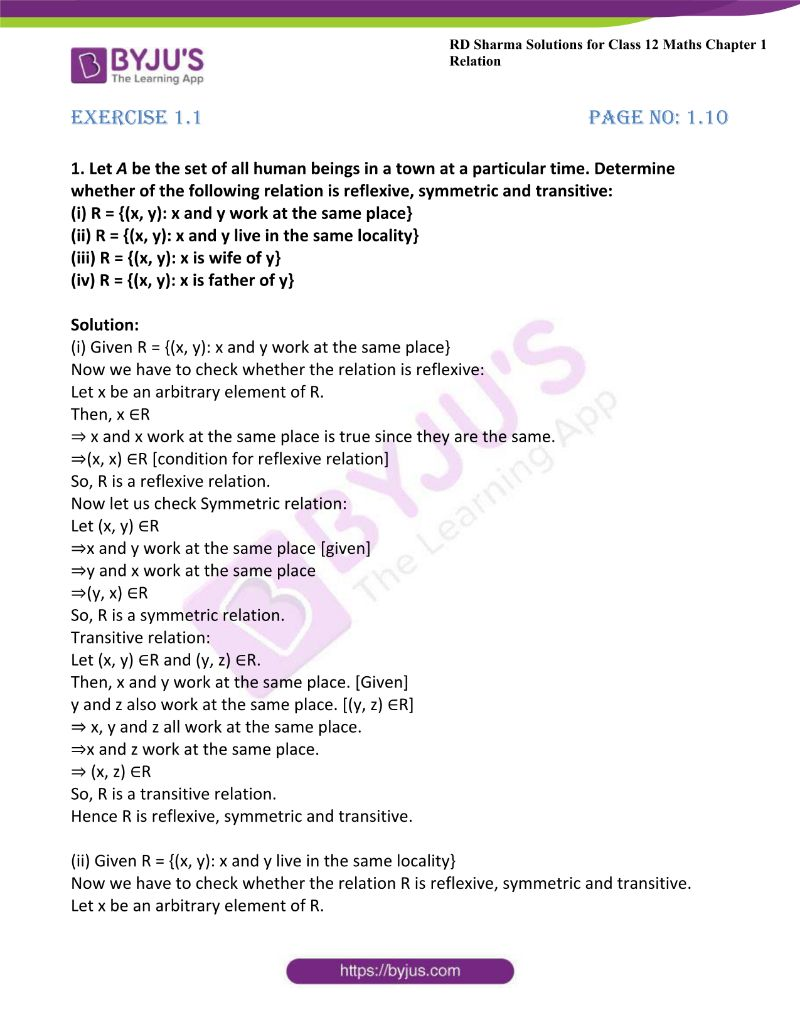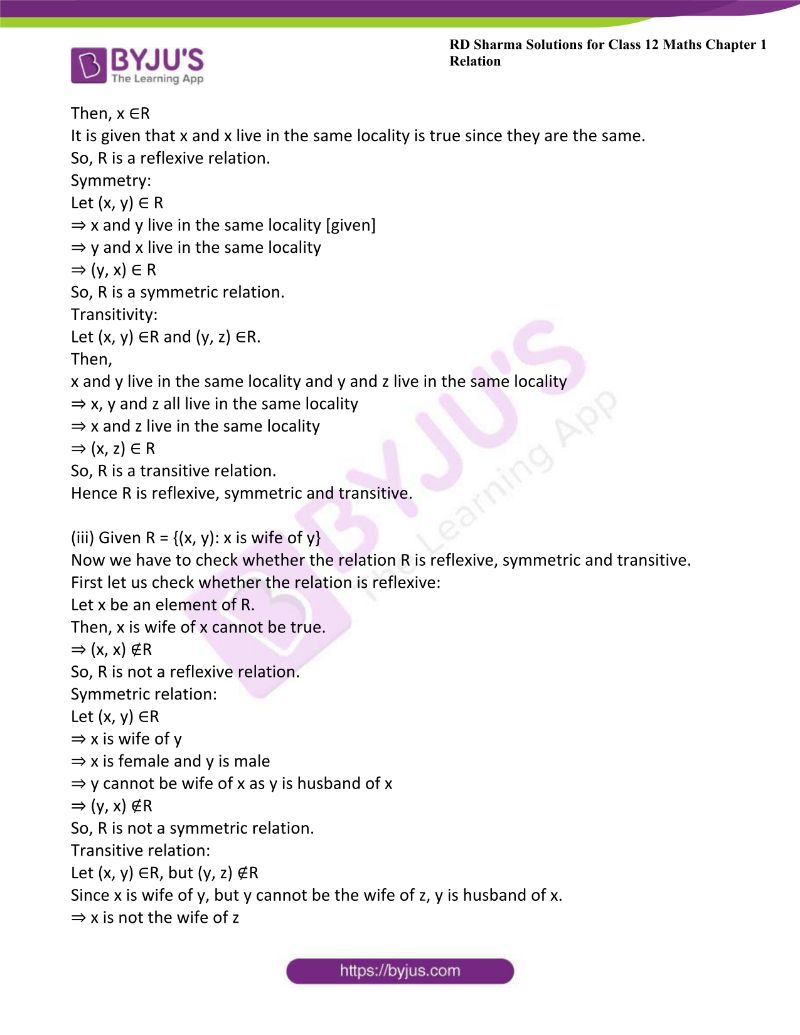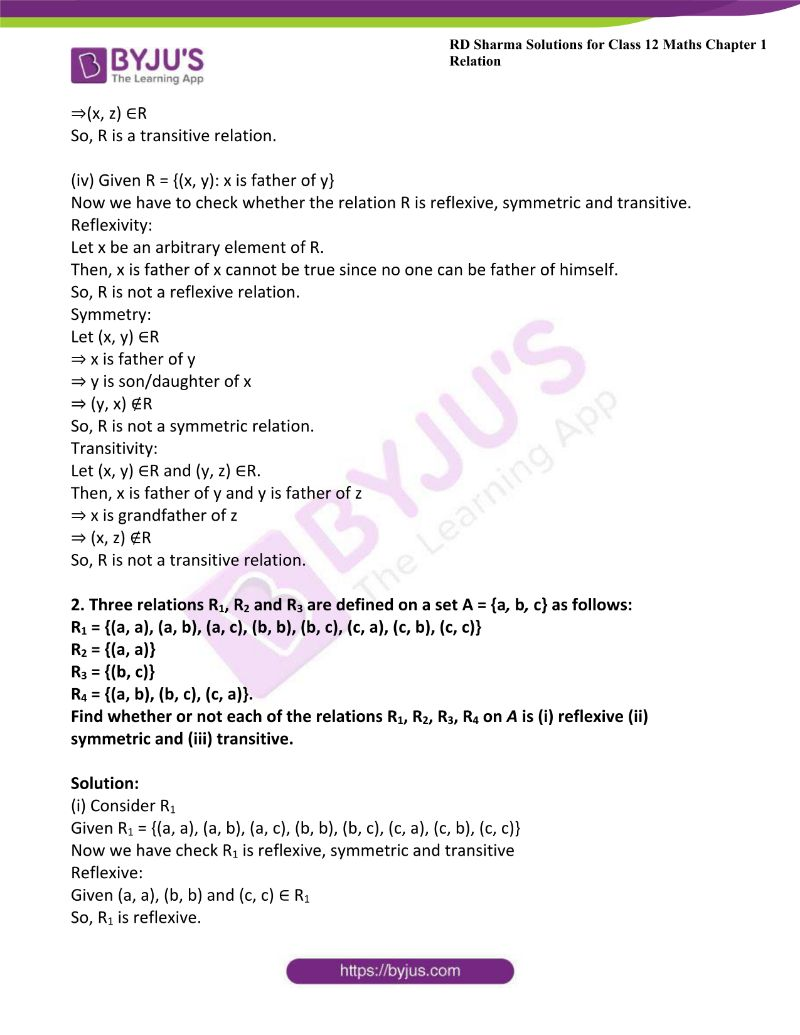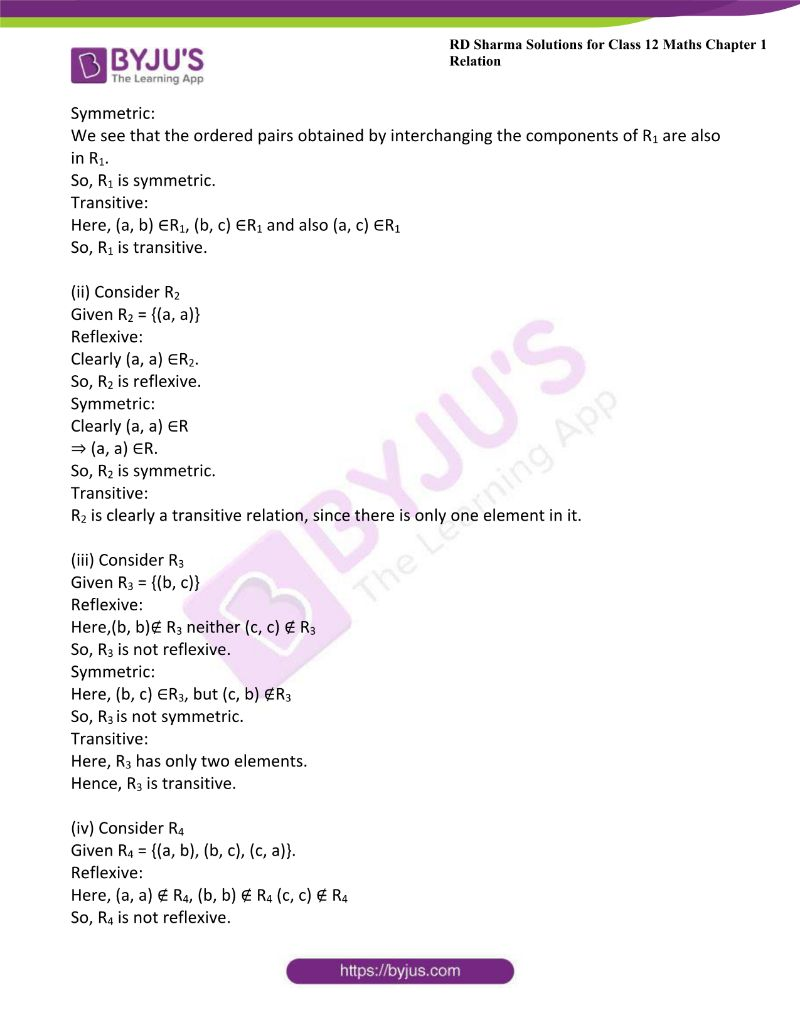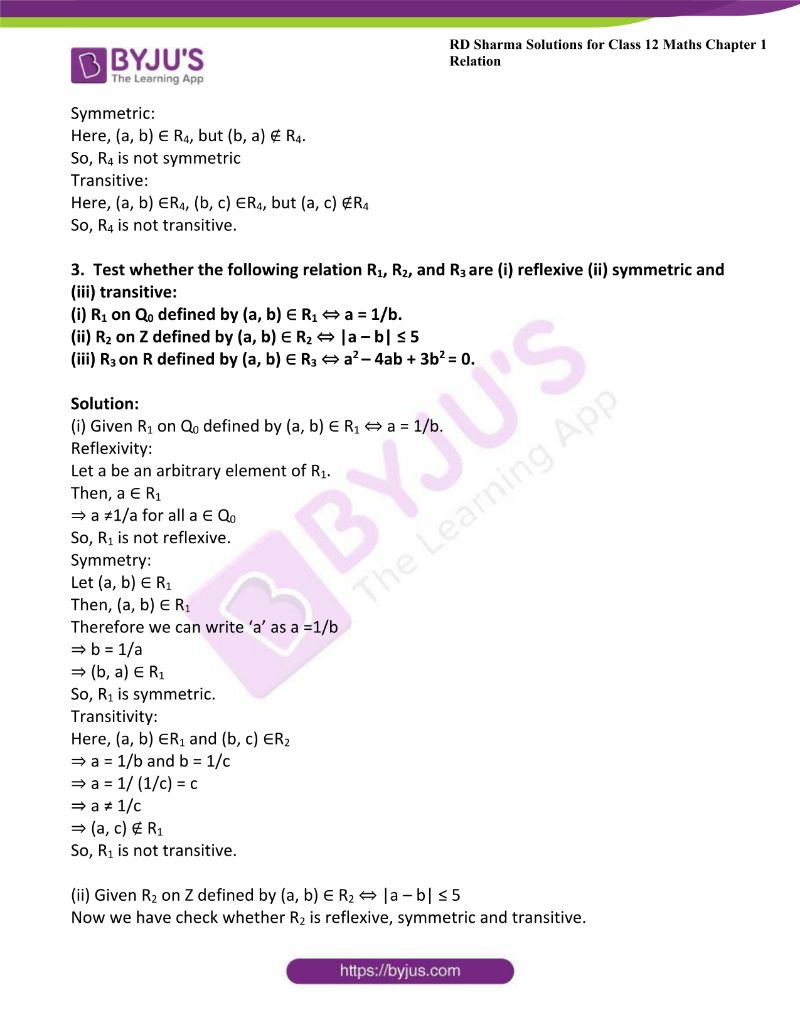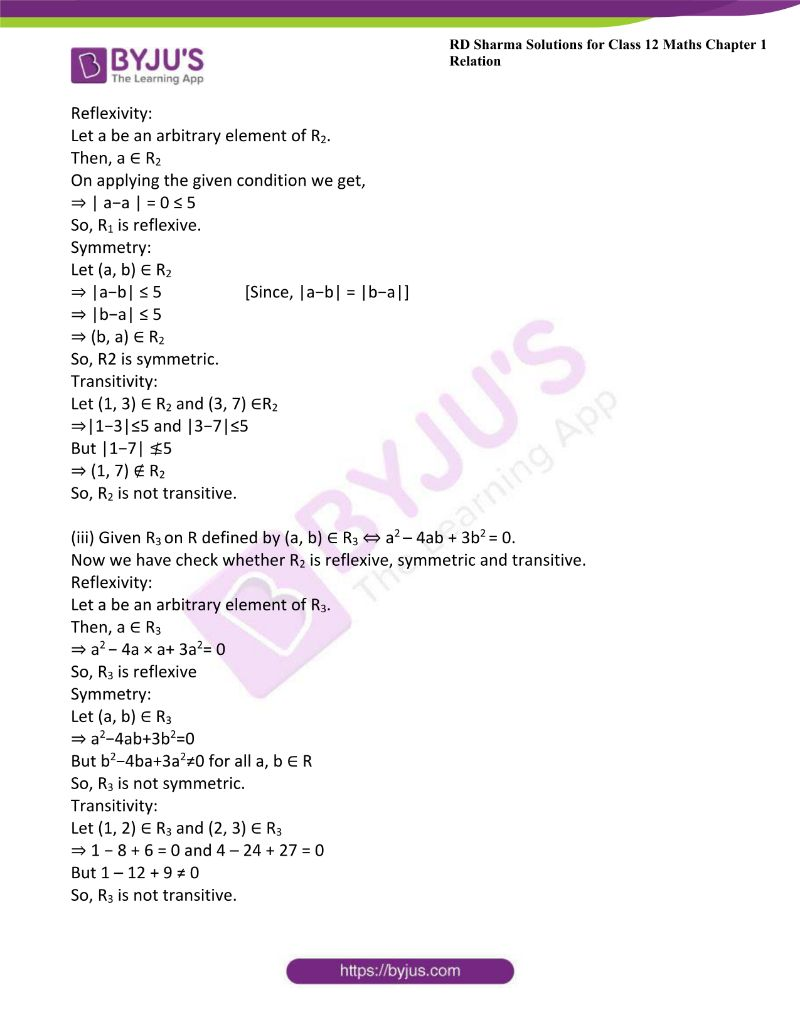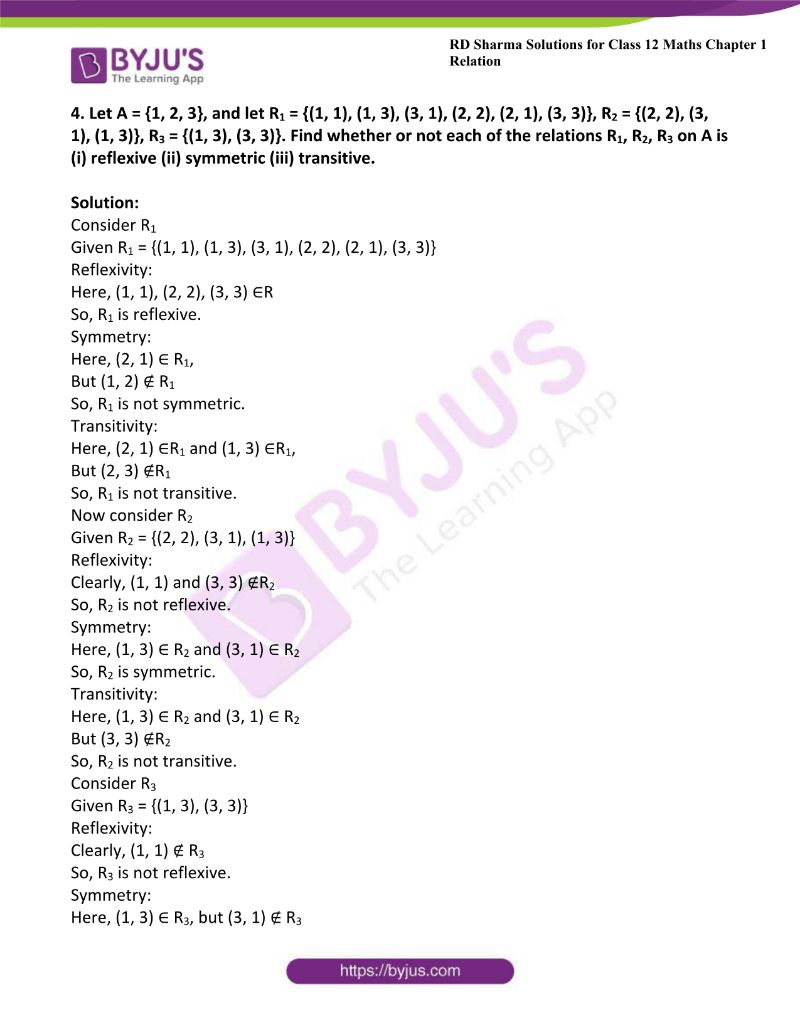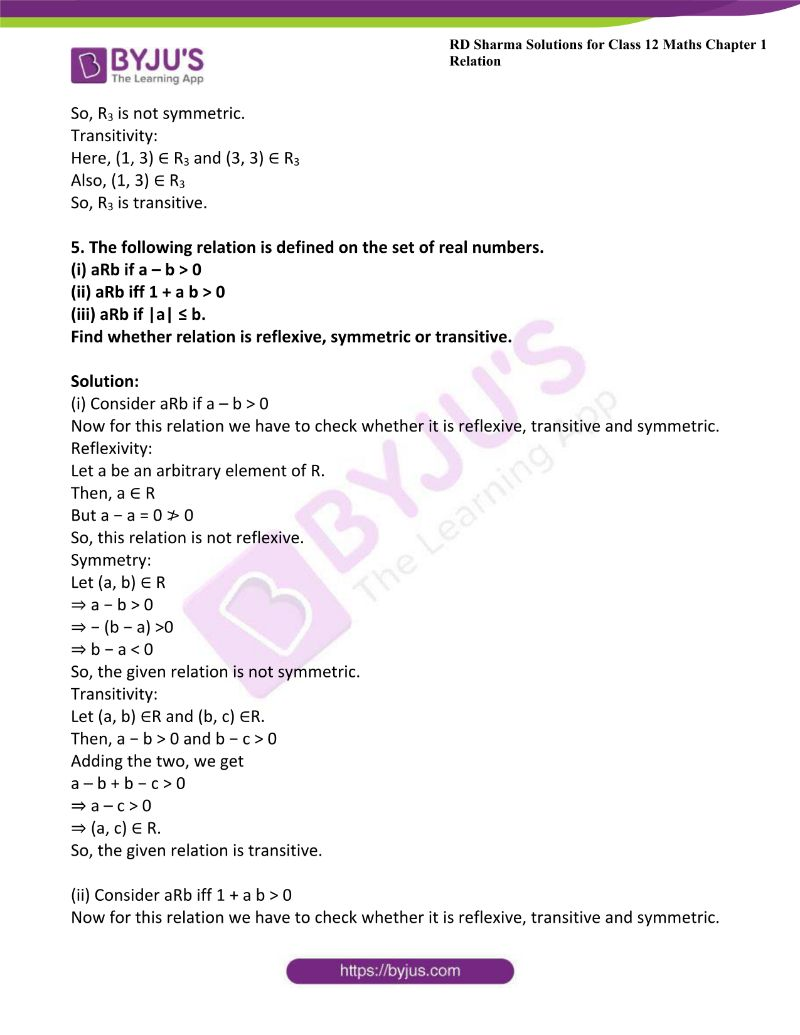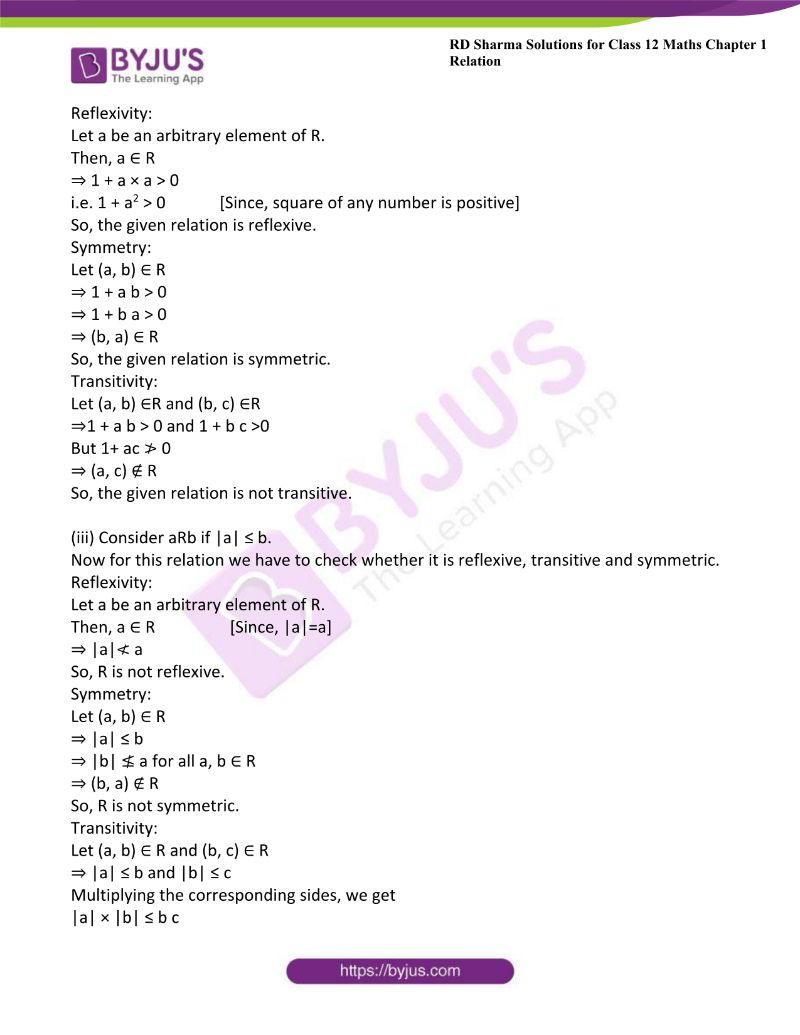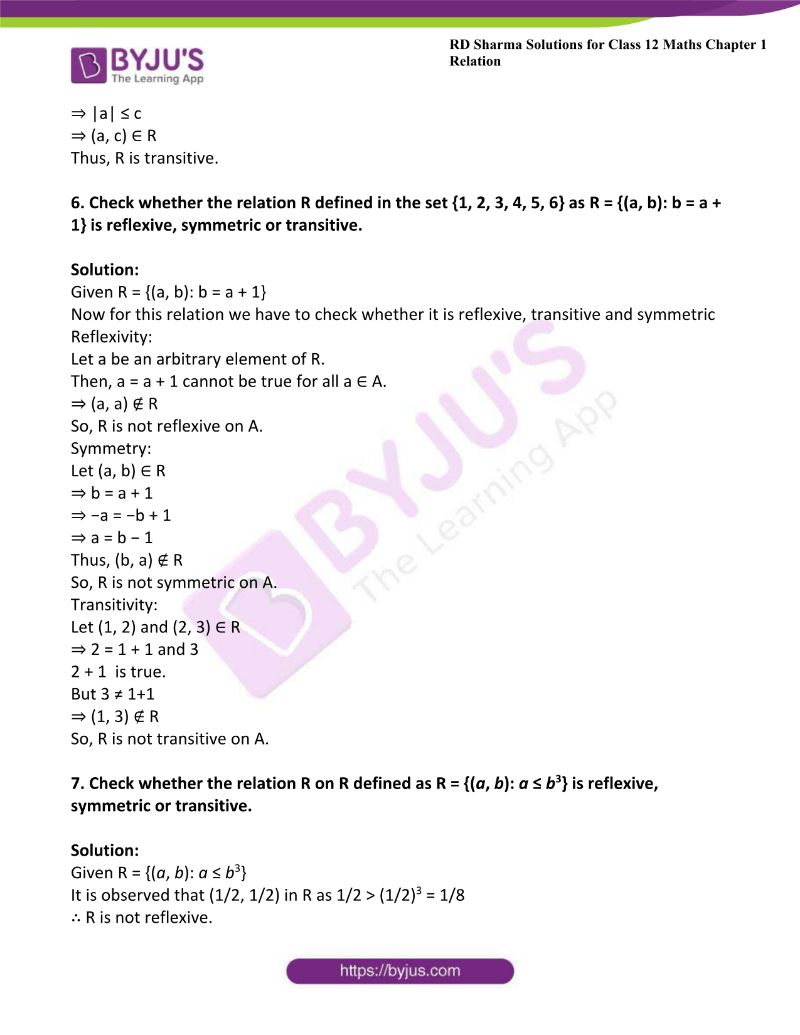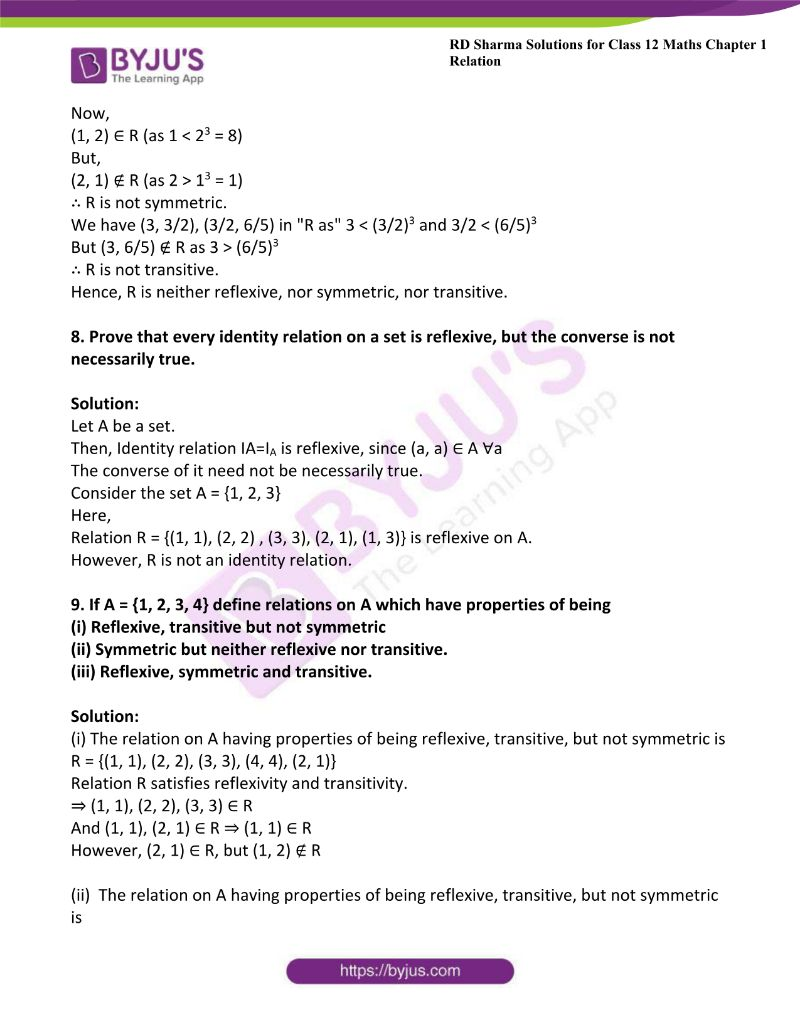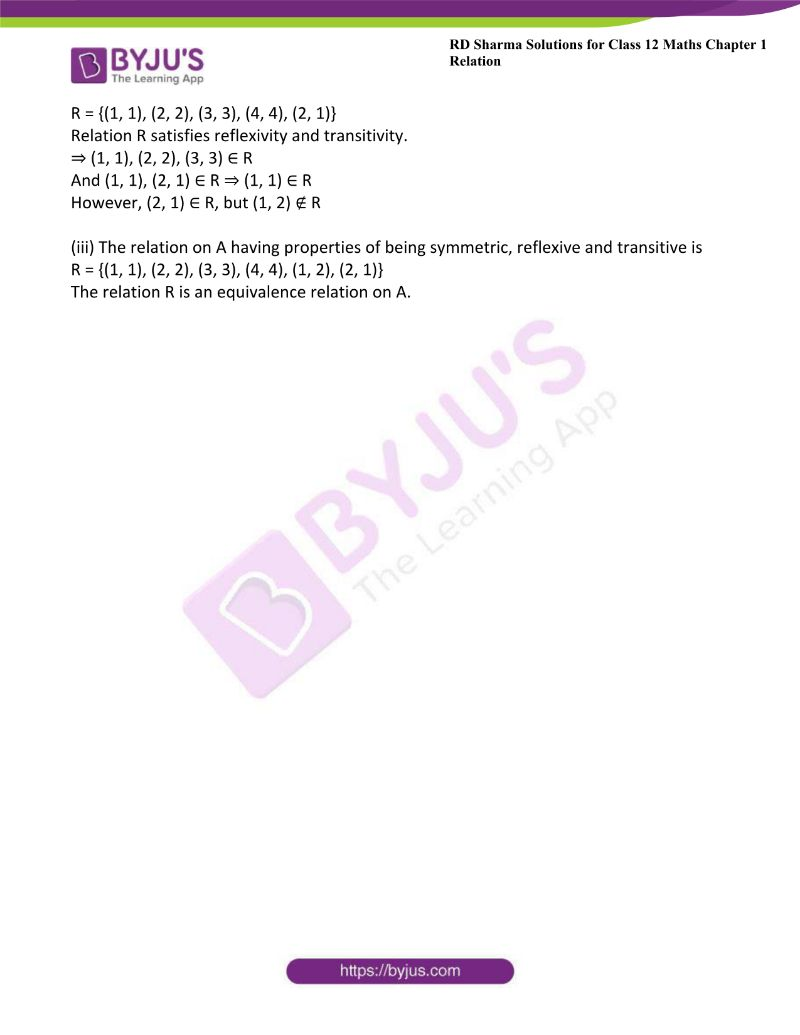### Access another exercise of RD Sharma Solutions For Class 12 Chapter 1 – Relations

Exercise 1.2 Solutions

### Access answers to Maths RD Sharma Solutions For Class 12 Chapter 1 – Relations Exercise 1.1

1. Let A be the set of all human beings in a town at a particular time. Determine whether of the following relation is reflexive, symmetric and transitive:

(i) R = {(x, y): x and y work at the same place}

(ii) R = {(x, y): x and y live in the same locality}

(iii) R = {(x, y): x is wife of y}

(iv) R = {(x, y): x is father of y}

Solution:

(i) Given R = {(x, y): x and y work at the same place}

Now we have to check whether the relation is reflexive:

Let x be an arbitrary element of R.

Then, x ∈R

⇒ x and x work at the same place is true since they are the same.

⇒(x, x) ∈R [condition for reflexive relation]

So, R is a reflexive relation.

Now let us check Symmetric relation:

Let (x, y) ∈R

⇒x and y work at the same place [given]

⇒y and x work at the same place

⇒(y, x) ∈R

So, R is a symmetric relation.

Transitive relation:

Let (x, y) ∈R and (y, z) ∈R.

Then, x and y work at the same place. [Given]

y and z also work at the same place. [(y, z) ∈R]

⇒ x, y and z all work at the same place.

⇒x and z work at the same place.

⇒ (x, z) ∈R

So, R is a transitive relation.

Hence R is reflexive, symmetric and transitive.

(ii) Given R = {(x, y): x and y live in the same locality}

Now we have to check whether the relation R is reflexive, symmetric and transitive.

Let x be an arbitrary element of R.

Then, x ∈R

It is given that x and x live in the same locality is true since they are the same.

So, R is a reflexive relation.

Symmetry:

Let (x, y) ∈ R

⇒ x and y live in the same locality [given]

⇒ y and x live in the same locality

⇒ (y, x) ∈ R

So, R is a symmetric relation.

Transitivity:

Let (x, y) ∈R and (y, z) ∈R.

Then,

x and y live in the same locality and y and z live in the same locality

⇒ x, y and z all live in the same locality

⇒ x and z live in the same locality

⇒ (x, z) ∈ R

So, R is a transitive relation.

Hence R is reflexive, symmetric and transitive.

(iii) Given R = {(x, y): x is wife of y}

Now we have to check whether the relation R is reflexive, symmetric and transitive.

First let us check whether the relation is reflexive:

Let x be an element of R.

Then, x is wife of x cannot be true.

⇒ (x, x) ∉R

So, R is not a reflexive relation.

Symmetric relation:

Let (x, y) ∈R

⇒ x is wife of y

⇒ x is female and y is male

⇒ y cannot be wife of x as y is husband of x

⇒ (y, x) ∉R

So, R is not a symmetric relation.

Transitive relation:

Let (x, y) ∈R, but (y, z) ∉R

Since x is wife of y, but y cannot be the wife of z, y is husband of x.

⇒ x is not the wife of z

⇒(x, z) ∈R

So, R is a transitive relation.

(iv) Given R = {(x, y): x is father of y}

Now we have to check whether the relation R is reflexive, symmetric and transitive.

Reflexivity:

Let x be an arbitrary element of R.

Then, x is father of x cannot be true since no one can be father of himself.

So, R is not a reflexive relation.

Symmetry:

Let (x, y) ∈R

⇒ x is father of y

⇒ y is son/daughter of x

⇒ (y, x) ∉R

So, R is not a symmetric relation.

Transitivity:

Let (x, y) ∈R and (y, z) ∈R.

Then, x is father of y and y is father of z

⇒ x is grandfather of z

⇒ (x, z) ∉R

So, R is not a transitive relation.

2. Three relations R1, R2 and R3 are defined on a set A = {a, b, c} as follows:
R1 = {(a, a), (a, b), (a, c), (b, b), (b, c), (c, a), (c, b), (c, c)}
R2 = {(a, a)}
R3 = {(b, c)}
R4 = {(a, b), (b, c), (c, a)}.

Find whether or not each of the relations R1, R2, R3, R4 on is (i) reflexive (ii) symmetric and (iii) transitive.

Solution:

(i) Consider R1

Given R1 = {(a, a), (a, b), (a, c), (b, b), (b, c), (c, a), (c, b), (c, c)}

Now we have check R1 is reflexive, symmetric and transitive

Reflexive:

Given (a, a), (b, b) and (c, c) ∈ R1

So, R1 is reflexive.

Symmetric:

We see that the ordered pairs obtained by interchanging the components of R1 are also in R1.

So, R1 is symmetric.

Transitive:

Here, (a, b) ∈R1, (b, c) ∈R1 and also (a, c) ∈R1

So, R1 is transitive.

(ii) Consider R2

Given R2 = {(a, a)}

Reflexive:

Clearly (a, a) ∈R2.

So, R2 is reflexive.

Symmetric:

Clearly (a, a) ∈R

⇒ (a, a) ∈R.

So, R2 is symmetric.

Transitive:

R2 is clearly a transitive relation, since there is only one element in it.

(iii) Consider R3

Given R3 = {(b, c)}

Reflexive:

Here,(b, b)∉ R3 neither (c, c) ∉ R3

So, R3 is not reflexive.

Symmetric:

Here, (b, c) ∈R3, but (c, b) ∉R3

So, R3 is not symmetric.

Transitive:

Here, R3 has only two elements.

Hence, R3 is transitive.

(iv) Consider R4

Given R4 = {(a, b), (b, c), (c, a)}.

Reflexive:

Here, (a, a) ∉ R4, (b, b) ∉ R4 (c, c) ∉ R4

So, R4 is not reflexive.

Symmetric:

Here, (a, b) ∈ R4, but (b, a) ∉ R4.

So, R4 is not symmetric

Transitive:

Here, (a, b) ∈R4, (b, c) ∈R4, but (a, c) ∉R4

So, R4 is not transitive.

3.  Test whether the following relation R1, R2, and R3 are (i) reflexive (ii) symmetric and (iii) transitive:

(i) R1 on Q0 defined by (a, b) ∈ R1 ⇔ a = 1/b.

(ii) R2 on Z defined by (a, b) ∈ R2 ⇔ |a – b| ≤ 5

(iii) Ron R defined by (a, b) ∈ R3 ⇔ a2 – 4ab + 3b2 = 0.

Solution:

(i) Given R1 on Q0 defined by (a, b) ∈ R1 ⇔ a = 1/b.

Reflexivity:

Let a be an arbitrary element of R1.

Then, a ∈ R1

⇒ a ≠1/a for all a ∈ Q0

So, R1 is not reflexive.

Symmetry:

Let (a, b) ∈ R1

Then, (a, b) ∈ R1

Therefore we can write ‘a’ as a =1/b

⇒ b = 1/a

⇒ (b, a) ∈ R1

So, R1 is symmetric.

Transitivity:

Here, (a, b) ∈R1 and (b, c) ∈R2

⇒ a = 1/b and b = 1/c

⇒ a = 1/ (1/c) = c

⇒ a ≠ 1/c

⇒ (a, c) ∉ R1

So, R1 is not transitive.

(ii) Given R2 on Z defined by (a, b) ∈ R2 ⇔ |a – b| ≤ 5

Now we have check whether R2 is reflexive, symmetric and transitive.

Reflexivity:

Let a be an arbitrary element of R2.

Then, a ∈ R2

On applying the given condition we get,

⇒ | a−a | = 0 ≤ 5

So, R1 is reflexive.

Symmetry:

Let (a, b) ∈ R2

⇒ |a−b| ≤ 5                    [Since, |a−b| = |b−a|]

⇒ |b−a| ≤ 5

⇒ (b, a) ∈ R2

So, R2 is symmetric.

Transitivity:

Let (1, 3) ∈ R2 and (3, 7) ∈R2

⇒|1−3|≤5 and |3−7|≤5

But |1−7| ≰5

⇒ (1, 7) ∉ R2

So, R2 is not transitive.

(iii) Given Ron R defined by (a, b) ∈ R3 ⇔ a2 – 4ab + 3b2 = 0.

Now we have check whether R2 is reflexive, symmetric and transitive.

Reflexivity:

Let a be an arbitrary element of R3.

Then, a ∈ R3

⇒ a− 4a × a+ 3a2= 0

So, R3 is reflexive

Symmetry:

Let (a, b) ∈ R3

⇒ a2−4ab+3b2=0

But b2−4ba+3a2≠0 for all a, b ∈ R

So, R3 is not symmetric.

Transitivity:

Let (1, 2) ∈ R3 and (2, 3) ∈ R3

⇒ 1 − 8 + 6 = 0 and 4 – 24 + 27 = 0

But 1 – 12 + 9 ≠ 0

So, R3 is not transitive.

4. Let A = {1, 2, 3}, and let R1 = {(1, 1), (1, 3), (3, 1), (2, 2), (2, 1), (3, 3)}, R2 = {(2, 2), (3, 1), (1, 3)}, R3 = {(1, 3), (3, 3)}. Find whether or not each of the relations R1, R2, R3 on A is (i) reflexive (ii) symmetric (iii) transitive.

Solution:

Consider R1

Given R1 = {(1, 1), (1, 3), (3, 1), (2, 2), (2, 1), (3, 3)}

Reflexivity:

Here, (1, 1), (2, 2), (3, 3) ∈R

So, R1 is reflexive.

Symmetry:

Here, (2, 1) ∈ R1,

But (1, 2) ∉ R1

So, R1 is not symmetric.

Transitivity:

Here, (2, 1) ∈R1 and (1, 3) ∈R1,

But (2, 3) ∉R1

So, R1 is not transitive.

Now consider R2

Given R2 = {(2, 2), (3, 1), (1, 3)}

Reflexivity:

Clearly, (1, 1) and (3, 3) ∉R2

So, R2 is not reflexive.

Symmetry:

Here, (1, 3) ∈ R2 and (3, 1) ∈ R2

So, R2 is symmetric.

Transitivity:

Here, (1, 3) ∈ R2 and (3, 1) ∈ R

But (3, 3) ∉R2

So, R2 is not transitive.

Consider R3

Given R3 = {(1, 3), (3, 3)}

Reflexivity:

Clearly, (1, 1) ∉ R3

So, R3 is not reflexive.

Symmetry:

Here, (1, 3) ∈ R3, but (3, 1) ∉ R3

So, R3 is not symmetric.

Transitivity:

Here, (1, 3) ∈ R3 and (3, 3) ∈ R3

Also, (1, 3) ∈ R3

So, R3 is transitive.

5. The following relation is defined on the set of real numbers.
(i) aRb if a – b > 0

(ii) aRb iff 1 + a b > 0

(iii) aRb if |a| ≤ b.

Find whether relation is reflexive, symmetric or transitive.

Solution:

(i) Consider aRb if a – b > 0

Now for this relation we have to check whether it is reflexive, transitive and symmetric.

Reflexivity:

Let a be an arbitrary element of R.

Then, a ∈ R

But a − a = 0 ≯ 0

So, this relation is not reflexive.

Symmetry:

Let (a, b) ∈ R

⇒ a − b > 0

⇒ − (b − a) >0

⇒ b − a < 0

So, the given relation is not symmetric.

Transitivity:

Let (a, b) ∈R and (b, c) ∈R.

Then, a − b > 0 and b − c > 0

a – b + b − c > 0

⇒ a – c > 0

⇒ (a, c) ∈ R.

So, the given relation is transitive.

(ii) Consider aRb iff 1 + a b > 0

Now for this relation we have to check whether it is reflexive, transitive and symmetric.

Reflexivity:

Let a be an arbitrary element of R.

Then, a ∈ R

⇒ 1 + a × a > 0

i.e. 1 + a2 > 0             [Since, square of any number is positive]

So, the given relation is reflexive.

Symmetry:

Let (a, b) ∈ R

⇒ 1 + a b > 0

⇒ 1 + b a > 0

⇒ (b, a) ∈ R

So, the given relation is symmetric.

Transitivity:

Let (a, b) ∈R and (b, c) ∈R

⇒1 + a b > 0 and 1 + b c >0

But 1+ ac ≯ 0

⇒ (a, c) ∉ R

So, the given relation is not transitive.

(iii) Consider aRb if |a| ≤ b.

Now for this relation we have to check whether it is reflexive, transitive and symmetric.

Reflexivity:

Let a be an arbitrary element of R.

Then, a ∈ R                  [Since, |a|=a]

⇒ |a|≮ a

So, R is not reflexive.

Symmetry:

Let (a, b) ∈ R

⇒ |a| ≤ b

⇒ |b| ≰ a for all a, b ∈ R

⇒ (b, a) ∉ R

So, R is not symmetric.

Transitivity:

Let (a, b) ∈ R and (b, c) ∈ R

⇒ |a| ≤ b and |b| ≤ c

Multiplying the corresponding sides, we get

|a| × |b| ≤ b c

⇒ |a| ≤ c

⇒ (a, c) ∈ R

Thus, R is transitive.

6. Check whether the relation R defined in the set {1, 2, 3, 4, 5, 6} as R = {(a, b): b = a + 1} is reflexive, symmetric or transitive.

Solution:

Given R = {(a, b): b = a + 1}

Now for this relation we have to check whether it is reflexive, transitive and symmetric Reflexivity:

Let a be an arbitrary element of R.

Then, a = a + 1 cannot be true for all a ∈ A.

⇒ (a, a) ∉ R

So, R is not reflexive on A.

Symmetry:

Let (a, b) ∈ R

⇒ b = a + 1

⇒ −a = −b + 1

⇒ a = b − 1

Thus, (b, a) ∉ R

So, R is not symmetric on A.

Transitivity:

Let (1, 2) and (2, 3) ∈ R

⇒ 2 = 1 + 1 and 3

2 + 1  is true.

But 3 ≠ 1+1

⇒ (1, 3) ∉ R

So, R is not transitive on A.

7. Check whether the relation R on R defined as R = {(ab): a ≤ b3} is reflexive, symmetric or transitive.

Solution:

Given R = {(ab): ≤ b3}

It is observed that (1/2, 1/2) in R as 1/2 > (1/2)3 = 1/8

∴ R is not reflexive.

Now,

(1, 2) ∈ R (as 1 < 23 = 8)

But,

(2, 1) ∉ R (as 2 > 13 = 1)

∴ R is not symmetric.

We have (3, 3/2), (3/2, 6/5) in “R as” 3 < (3/2)3 and 3/2 < (6/5)3

But (3, 6/5) ∉ R as 3 > (6/5)3

∴ R is not transitive.

Hence, R is neither reflexive, nor symmetric, nor transitive.

8. Prove that every identity relation on a set is reflexive, but the converse is not necessarily true.

Solution:

Let A be a set.

Then, Identity relation IA=IA is reflexive, since (a, a) ∈ A ∀a

The converse of it need not be necessarily true.

Consider the set A = {1, 2, 3}

Here,

Relation R = {(1, 1), (2, 2) , (3, 3), (2, 1), (1, 3)} is reflexive on A.

However, R is not an identity relation.

9. If A = {1, 2, 3, 4} define relations on A which have properties of being

(i) Reflexive, transitive but not symmetric

(ii) Symmetric but neither reflexive nor transitive.

(iii) Reflexive, symmetric and transitive.

Solution:

(i) The relation on A having properties of being reflexive, transitive, but not symmetric is

R = {(1, 1), (2, 2), (3, 3), (4, 4), (2, 1)}

Relation R satisfies reflexivity and transitivity.

⇒ (1, 1), (2, 2), (3, 3) ∈ R

And (1, 1), (2, 1) ∈ R ⇒ (1, 1) ∈ R

However, (2, 1) ∈ R, but (1, 2) ∉ R

(ii)  The relation on A having properties of being reflexive, transitive, but not symmetric is

R = {(1, 1), (2, 2), (3, 3), (4, 4), (2, 1)}

Relation R satisfies reflexivity and transitivity.

⇒ (1, 1), (2, 2), (3, 3) ∈ R

And (1, 1), (2, 1) ∈ R ⇒ (1, 1) ∈ R

However, (2, 1) ∈ R, but (1, 2) ∉ R

(iii) The relation on A having properties of being symmetric, reflexive and transitive is

R = {(1, 1), (2, 2), (3, 3), (4, 4), (1, 2), (2, 1)}

The relation R is an equivalence relation on A.

#### 1 Comment

1. sahil slathia

lots of love to this app